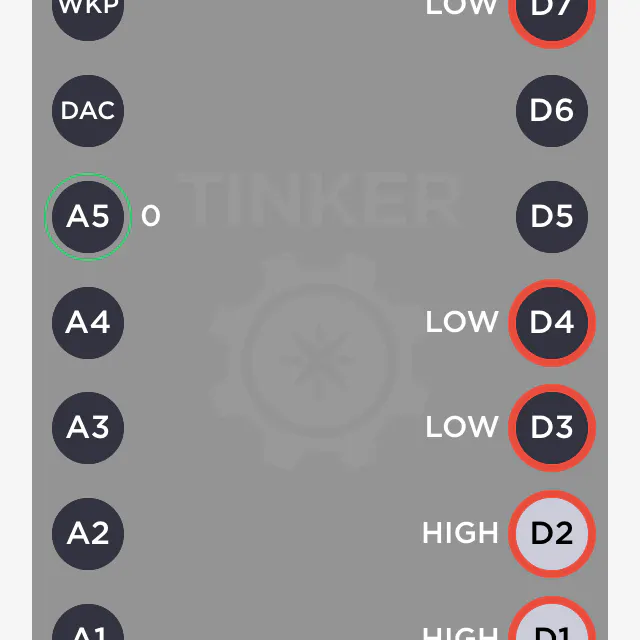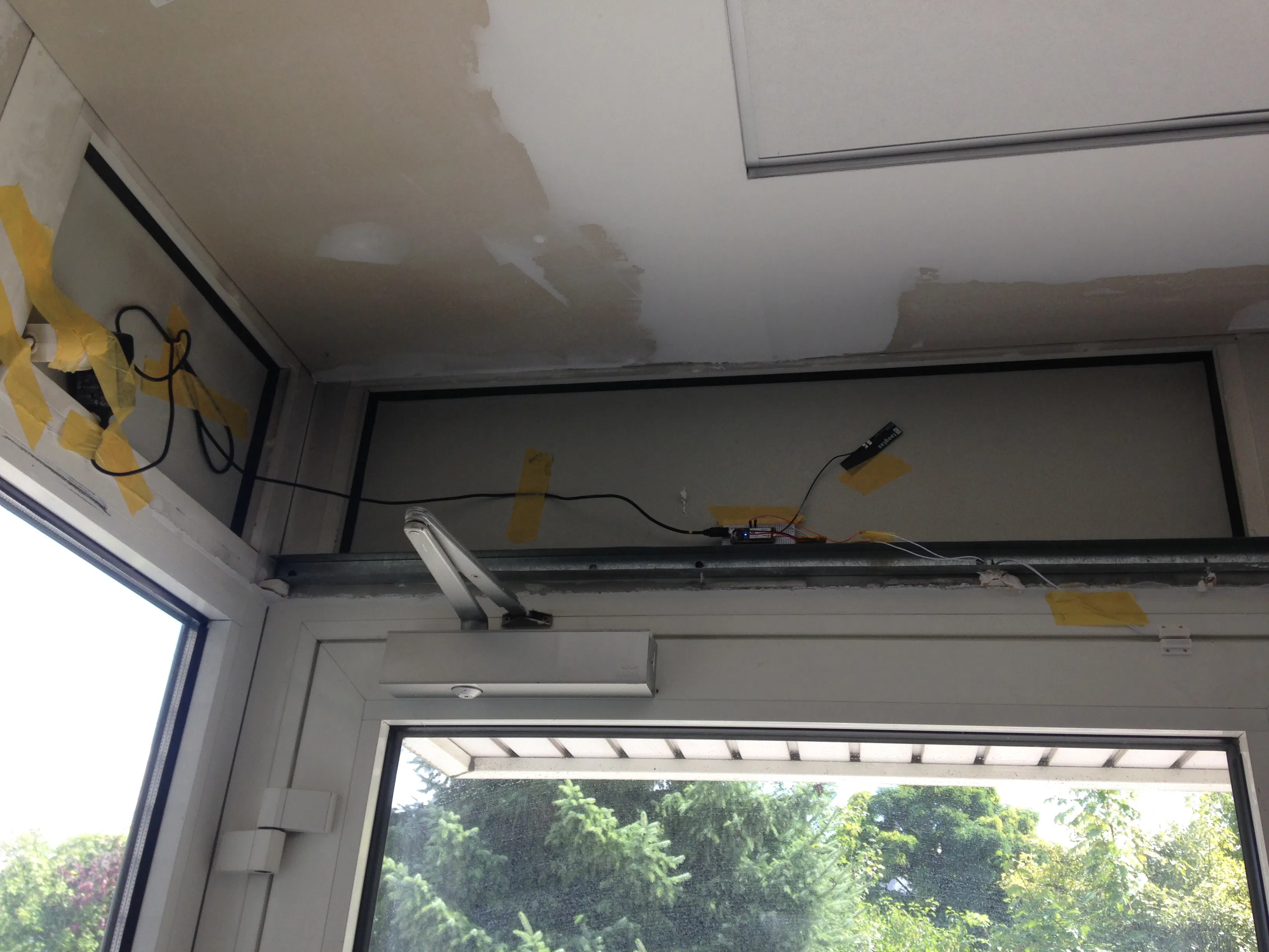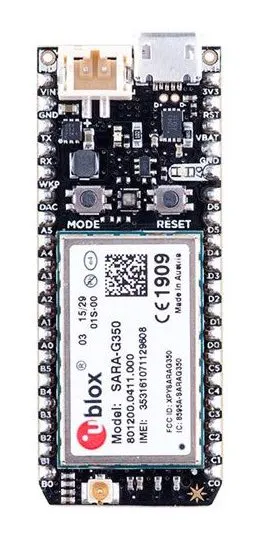# MyReed-Switch Controlled by Particle Electron

Magnetism in a simple door monitoring.

BeginnerFull instructions provided1 hour812## Things used in this project

### Hardware componentsParticle Electron
×1×1Jumper wires (generic) 2 pieces
×1
 Reed-Switch Magnetic Reed Switch with Adhesive
×1

### Software apps and online services

 WebIDEIFTTT Maker service

## Code

### MyReed-Switch

C/C++
v1.0
```// Reed-Switch with Particle Electron - works with v0.6.0 without Libaries
// Ingo Lohs, v1.0 v. 04.08.2017
// Projekt, um eine Eingangstür des Nachts zu überwachen
// Signalisierung erfolgt via IFTTT an Empfänger bei Trigger des Events

const int REED_PIN_IN = D2; // Pin connected to reed switch
const int REED_PIN_OUT = D4; // Pin connected to reed switch
const int LED_PIN = D7; // LED pin - active-high
bool firstfire = true;
// gewünschtes Überwachungszeitfenster - in der Regel über Nacht
int start_h = 22;
int ende_h = 7;

void setup()
{
Serial.begin(9600);
Particle.publish("EMF-Bielefeld - Eingangstür Projekt - Setup");
// Since the other end of the reed switch is connected to ground, we need
// to pull-up the reed switch pin internally.
pinMode(REED_PIN_IN, INPUT);
pinMode(REED_PIN_OUT, OUTPUT);
pinMode(LED_PIN, OUTPUT);

// Now flash the D7 LED on and off
digitalWrite(LED_PIN,HIGH); // Start der Kallibrierung mit D7 on
Particle.publish("EMF-Bielefeld - Eingangstür Projekt","now online",100,PRIVATE);
digitalWrite(LED_PIN,LOW); // Start der Kallibrierung mit D7 off

// setup a time zone, which is part of the ISO6801 format
Time.zone(+2.00);
}

void loop()
{

int h = Time.hour();
//Serial.print("aktuelle Stunde: ");
//Serial.println(h);

// prüft nicht den Wochentag ab, sondern schlicht die Stunde
if ((h >= start_h /*22*/) and (h <= 24) or (h >= 0) and (h <= ende_h /*7*/))
{

if ((proximity == HIGH) and (firstfire == true)) // If the pin reads HIGH, the switch is open // the pin reads LOW, the switch is closed
{
Serial.println(">> Alarm >> Switch open");
digitalWrite(LED_PIN, HIGH); // Turn the LED on
Particle.publish("EMF-Bielefeld - Eingangstür Projekt", "Tür ist offen", PRIVATE);
firstfire = false;
delay(1000);
}
else if ((proximity == HIGH) and (firstfire == false)) // If the pin reads HIGH, the switch is open.
{
Serial.println("Switch is open a while");
digitalWrite(LED_PIN, HIGH); // Turn the LED off
firstfire = false;
delay(1000);
}
else if ((proximity == LOW) and (firstfire == true))
{
Serial.println("Switch closed");
digitalWrite(LED_PIN, LOW); // Turn the LED off
firstfire = true;
delay(1000);
}
else if ((proximity == LOW) and (firstfire == false))
{
Serial.println("Switch closed");
digitalWrite(LED_PIN, LOW); // Turn the LED off
firstfire = true;
delay(1000);
}
else
{
}
}
else
{
Serial.print("keine Überwachung - nur von ");
Serial.print(start_h);
Serial.print(" bis ");
Serial.print(ende_h);
Serial.println(" Uhr!");
delay(2000);
}

}
```

### MyReed-Switch

C/C++
v1.1
```// Reed-Switch with Particle Electron - works with v0.6.0 without Libaries
// Ingo Lohs, v1.0 v. 04.08.2017
// Projekt, um eine Eingangstür des Nachts zu überwachen
// Signalisierung erfolgt via IFTTT an Empfänger bei Trigger des Events
// v1.1 - Umstellung des REED_PIN_IN von Input zu Input_Pullup > so bleibt der Zustand erhalten - gab sonst Probleme mit IFTTT
//      - Streichung der Delays unter Aufnahme von lastmillis bei Auslagerung in die Funktion "readData()"

const int REED_PIN_IN = D2; // Pin connected to reed switch
const int REED_PIN_OUT = D4; // Pin connected to reed switch
const int LED_PIN = D7; // LED pin - active-high
bool firstfire = true;
// gewünschtes Überwachungszeitfenster - in der Regel über Nacht
int start_h = 22;
int ende_h = 7;

unsigned long lastmillis = 0; // time for interation the loop

void setup()
{
Serial.begin(9600);
Particle.publish("EMF-Bielefeld - Eingangstür Projekt - Setup");
// Since the other end of the reed switch is connected to ground, we need
// to pull-up the reed switch pin internally.
pinMode(REED_PIN_IN, INPUT_PULLUP);
pinMode(REED_PIN_OUT, OUTPUT);
pinMode(LED_PIN, OUTPUT);

// Now flash the D7 LED on and off
digitalWrite(LED_PIN,HIGH); // Start der Kallibrierung mit D7 on
Particle.publish("EMF-Bielefeld - Eingangstür Projekt","now online",100,PRIVATE);
digitalWrite(LED_PIN,LOW); // Start der Kallibrierung mit D7 off

// setup a time zone, which is part of the ISO6801 format
Time.zone(+2.00);
}

void loop()
{
if ((millis() - lastmillis) > 2000) {
lastmillis = millis();
}
}

int h = Time.hour();
//Serial.print("aktuelle Stunde: ");
//Serial.println(h);

// prüft nicht den Wochentag ab, sondern schlicht die Stunde
if ((h >= start_h /*22*/) and (h <= 24) or (h >= 0) and (h <= ende_h /*7*/))
{

if ((proximity == HIGH) and (firstfire == true)) // If the pin reads HIGH, the switch is open // the pin reads LOW, the switch is closed
{
Serial.println(">> Alarm >> Switch open");
digitalWrite(LED_PIN, HIGH); // Turn the LED on
Particle.publish("EMF-Bielefeld - Eingangstür Projekt", "Tür ist offen", PRIVATE);
firstfire = false;
}
else if ((proximity == HIGH) and (firstfire == false)) // If the pin reads HIGH, the switch is open.
{
Serial.println("Switch is open a while");
digitalWrite(LED_PIN, HIGH); // Turn the LED on
firstfire = false;
}
else if ((proximity == LOW) and (firstfire == true))
{
Serial.println("Switch closed");
digitalWrite(LED_PIN, LOW); // Turn the LED off
firstfire = true;
}
else if ((proximity == LOW) and (firstfire == false))
{
Serial.println("Switch closed");
digitalWrite(LED_PIN, LOW); // Turn the LED off
firstfire = true;
}
else
{
}
}
else
{
Serial.print("keine Überwachung - nur von ");
Serial.print(start_h);
Serial.print(" bis ");
Serial.print(ende_h);
Serial.println(" Uhr!");
}

}
```

## Credits

### Ingo Lohs

48 projects • 193 followers
I am well over 50 years and come from the middle of Germany.